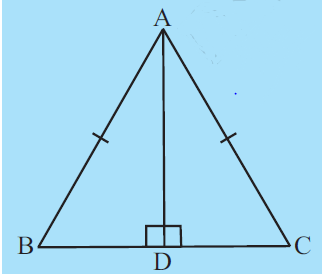Q

# ABC is an isosceles triangle with AB = AC and AD is one of its altitudes (Fig 7.34). State the three pairs of equal parts in bigtriangleup ADB and bigtriangleup ADC. Is bigtriangleup ADB cong bigtriangleup ADC? Why or why not?

4. ABC is an isosceles triangle with and is one of its altitudes (Fig 7.34).

(i) State the three pairs of equal parts in and .
(ii) Is ? Why or why not?
(iii) Is ? Why or why not?
(iv) Is ? Why or why not?Views

i) Given in and .

( Common side)

ii) So, by RHS Rule of congruency, we conclude

iii) Since both triangles are congruent all the corresponding parts will be equal.

So,

iv) Since both triangles are congruent all the corresponding parts will be equal.

So,

.

Exams
Articles
Questions